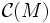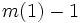# Connection is module structure over connection algebra

(diff) ← Older revision | Latest revision (diff) | Newer revision → (diff)

## Statement

Let$E$ be a vector bundle over a differential manifold$M$. Then, a connection on$E$ is equivalent to giving$E$ the structure of a module over the connection algebra over$M$.

## Definitions used

### Connection

Further information: Connection

### Connection algebra

Further information: Connection algebra

## Proof

We start with a connection$\nabla$ on$E$ and show how$\nabla$ naturally equips$E$ with the structure of a module over$\mathcal{C}(M)$.

First, observe that a connection gives a rule for the Lie algebra of first-order differential operators to act on$E$, hence the tensor algebra generated by it as a vector space, acts on$E$. We need to check that under this action$m(1) - 1$ acts trivially on$E$.